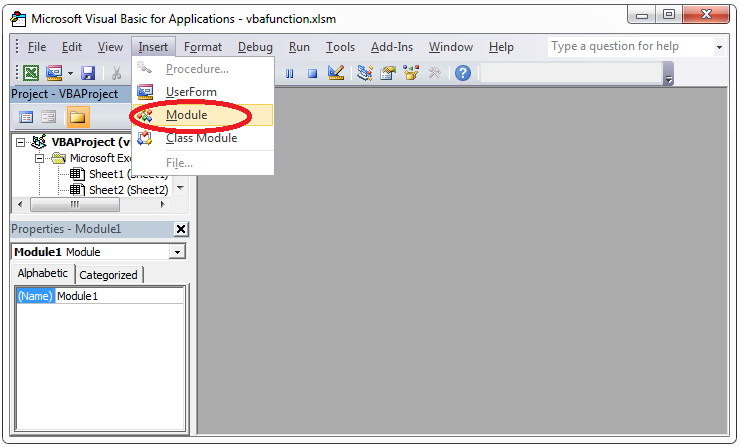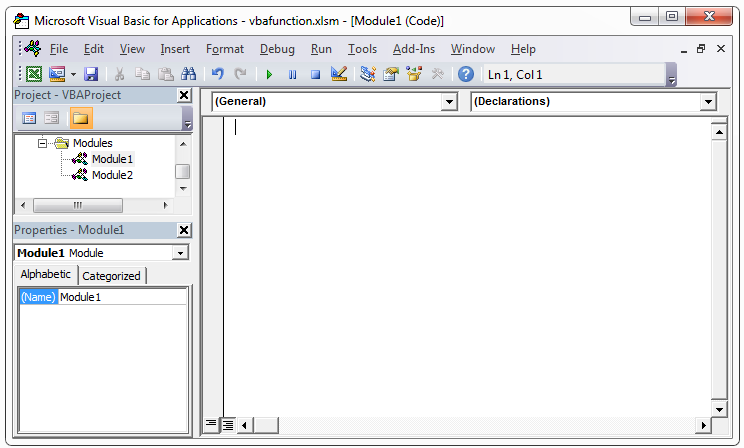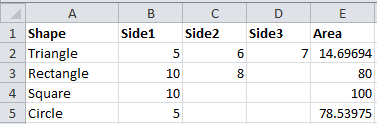### How to Create Your Own VBA Function in Excel

Excel offers a large number of built-in functions which you can use to perform many different tasks easily and quickly. But at least once, you might have felt that the built-in functions provided by Excel are not enough for you to perform some complicated task. In such cases, you will have to write your own functions and this is done using VBA. VBA stands for Visual Basic for Applications. Moreover, whenever you have to use a block of code repeatedly, it is advisable to create a function so that you can reuse the code easily.

For example, you want to calculate the area of different shapes like rectangle, square, circle, triangle etc. If you check the built-in formulas, you could not find any formula for the area calculation. Let’s do it by creating a function.

### Create a VBA function

Step 1. Open Excel and save your file as vbafunction.xlsm. Make sure you save the file as Microsoft Excel Macro-Enabled Worksheet. Otherwise, the function you are going to create will not work.

Step 2. Type “Shape” in A1, “Side1” in B1, “Side2” in C1, “Side3” in D1, “Area” in E1, “Triangle” in A2, “Rectangle” in A3, “Square” in A4 and “Circle” in A5. You can format the cells A1, B1, C1, D1 and E1 and make them bold. Now your screen will look like this:Step 3. Click ALT + F11 to open the Visual Basic Editor.Step 4. Go to Insert –> Module.Now you will get a screen like this:This is the area where you need to enter your functions.

Step 5. Enter the following code in the space provided:

Function AreaRectangle(Height As Double, Width As Double) As Double

AreaRectangle = Width * Height

End Function

Function AreaTriangle(Side1 As Double, Side2 As Double, Side3 As Double) As Double

Dim p As Double

p = (Side1 + Side2 + Side3) / 2

AreaTriangle = Sqr(p * (p – Side1) * (p – Side2) * (p – Side3))

End Function

Function AreaSquare(Side As Double) As Double

AreaSquare = Side * Side

End Function

Function AreaCircle(Radius As Double) As Double

End Function

Now your screen will look like this:Step 6. Save these functions by clicking the Save icon or by going to File –> Save vbafunction.xlsm or by clicking CTRL + S.

Step 7. Click ALT + F11 to go back to your Excel sheet.

Step 8. Type 5, 6 and 7 in the cells B2, C2 and D2. Type 10 and 8 in the cells B3 and D3. Type 10 in the cell B4. Type 5 in the cell B5. Now your screen will look like this:Step 9. Click the cell E2 and go to the formula bar and enter =ar. When you enter the first few lines of the name of your function, you could find that your function is listed along with other Excel built-in functions like this:Select your function from the list or enter the function name completely as =areaTriangle(B2,C2,D2) and press Enter key. Here B2, C2 and D2 are the cells that contain the three sides of the triangle. Now the cell E2 will contain the value 14.69694.

Step 10. Click the cell E3 and enter the formula =areaRectangle(B3,C3). The cell E3 will have the value 80.

Step 11. Click the cell E4 and enter the formula =areaSquare(B4). The cell E4 will have the value 100.

Step 12. Click the cell E5 and enter the formula =areaCircle(B5). Te cell E5 will have the value 78.53975. Now your screen will look like this:Here, you have created simple mathematical functions to calculate areas of different shapes. Likewise, you can create more complicated functions. You can even use built-in Excel functions in your functions and make the code reusable.

## Template

```Further reading: